# How to Calculate Days Between Two Dates in Excel

As an Excel user, there may be times when you need to add start and end date columns to your spreadsheets. As such, Excel includes a few functions that tell you how many days there are between two separate dates. You can add four Excel functions to cells that tell you how many days exist between two specified dates. This article describes using Excel to calculate days between two selected dates with and without using those functions. Let’s get started.## How to Find the Difference Between Dates in Excel without a Function

First, you can find the difference between dates by subtracting them. Excel doesn’t include a subtract function, but you can still add subtraction formulas to your cells. Here’s how to do it for dates.

1. Open a “blank” Excel spreadsheet, and enter a “start” and “end” date in cells “B4” and “C4,” as in the snapshot below. Note that the dates should be in U.S. format with the month first, the day second, and the year third.2. Now, select cell “D4,” click inside the “formula bar” at the top, then type “`=C4-B4`” and press “Enter.” Cell “D4” will return a value of “34.” Note: “C4” comes first because you are subtracting.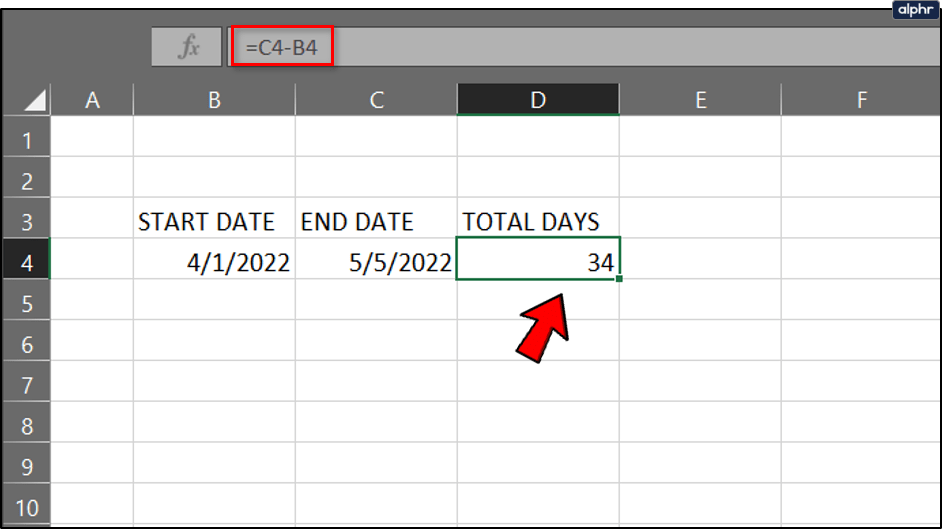## How to Find the Difference Between Two Dates in Excel using the DATE Function

You can find the difference between any two dates using the DATE function. Then, you can find the number of days by entering the dates in the function bar instead of spreadsheet cells. The basic syntax for that function is “=DATE(yyyy, m, d)-DATE(yyyy, m, d).” To correctly calculate the difference, the latest date gets inserted first.

1. Select a “cell” on the spreadsheet where you want to add the function, then ensure it is set to “General” format.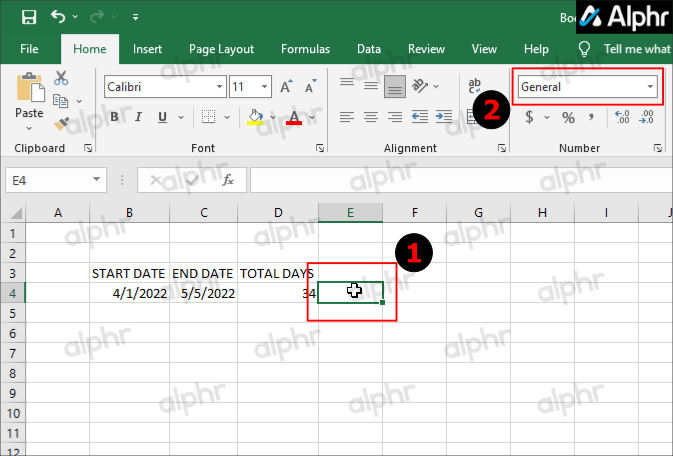2. Click inside the “function bar,” then type in “`=DATE(2022, 5, 5)-DATE(2022, 4, 1)`” and press “Enter.”## Finding the Difference Between Two Dates using the DATEDIF Function

DATEDIF is a flexible function to calculate the total days by entering dates on the spreadsheet or in the function bar. However, DATEDIF is not listed on Excel’s Insert Function window because it only exists for Lotus 1-2-3 workbook compatibility.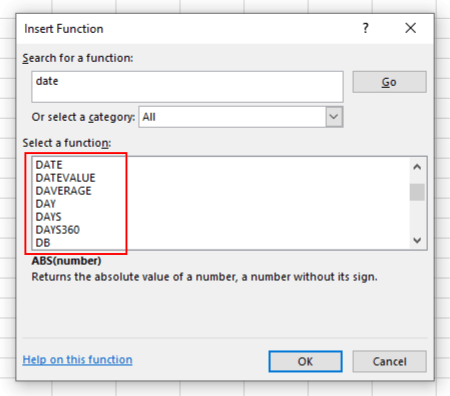Also, the use of DATEDIF may produce incorrect results in some situations. For more details, see this Excel DATEIF help page.

If you decide to use the “DATEIF” function, you’ll need to enter it directly in the function bar. The syntax includes DATEDIF(start_date, end_date, unit). You can enter a start date and end date or cell references to specific dates in the function and then add the unit “days” to the end of it. Here’s how to do it.

1. Select the “cell” on the spreadsheet where you want to place the function, then set it to “General” format.2. To find the difference in days (including years) entered in cells B6 and C6, type in “`=DATEDIF(B6, C6, "d")`” into the function bar and press “Enter.” The “d” represents the format “days.”3. If you need to ignore years in the calculation, replace “d” with “yd,” so you get a formula listed as “`=DATEDIF(B4, C4, "yd")`.” The “y” excludes years, but the “d” includes “days.”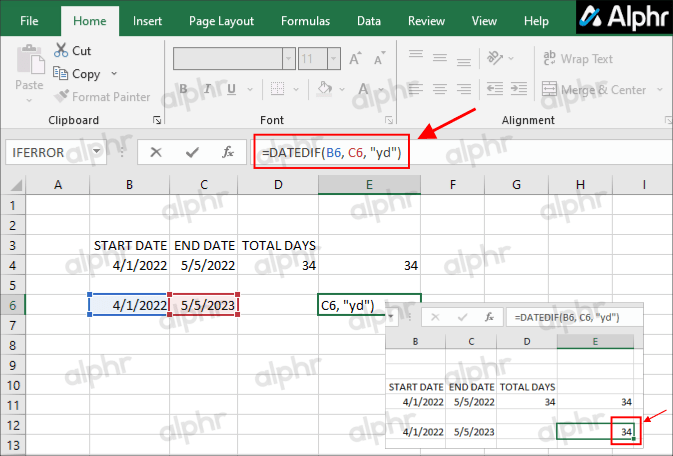If you encounter any errors, insert the earliest cell, listed as “B6,” in the example above.

## Finding the Difference Between Two Dates using the DAYS360 Function

The DAYS360 function finds the total days between dates based on a 360-day calendar, which is more widely adopted for financial years. As such, that might be a better function for account spreadsheets. It won’t make much difference for dates just a few months apart, but DAYS360 will return slightly different values for more extended periods than the other functions.

1. Enter ‘1/1/2021‘ and ‘1/1/2022‘ in cells B6 and C6 on your spreadsheet.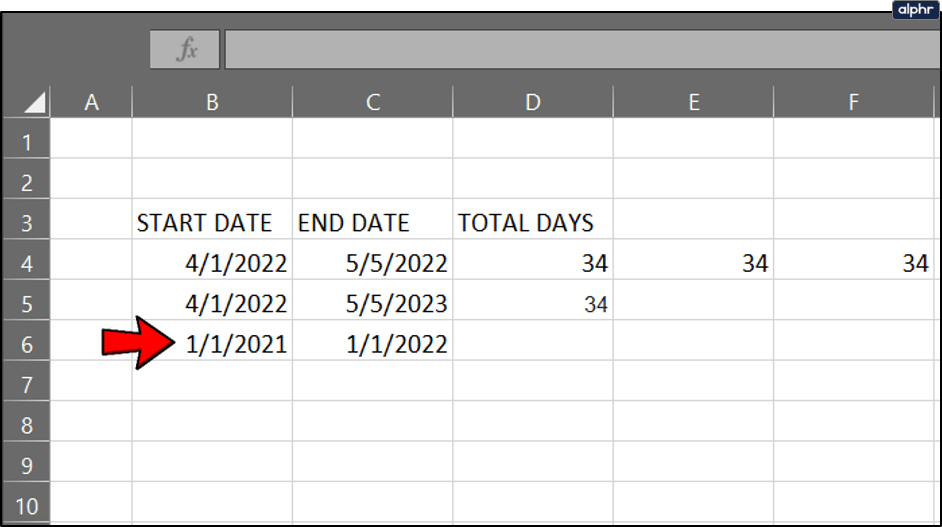2. Then click a cell to include the “DAYS360” function, and click “Formulas > Date & Time.”3. From the “Date & Time” dropdown menu, select “DAYS360.”4. Click the “Start_date” button and type in “B6,” click the “End_date” button and type in “C6,” and then press “OK.”5. The “DAYS360” function will return a value of 360.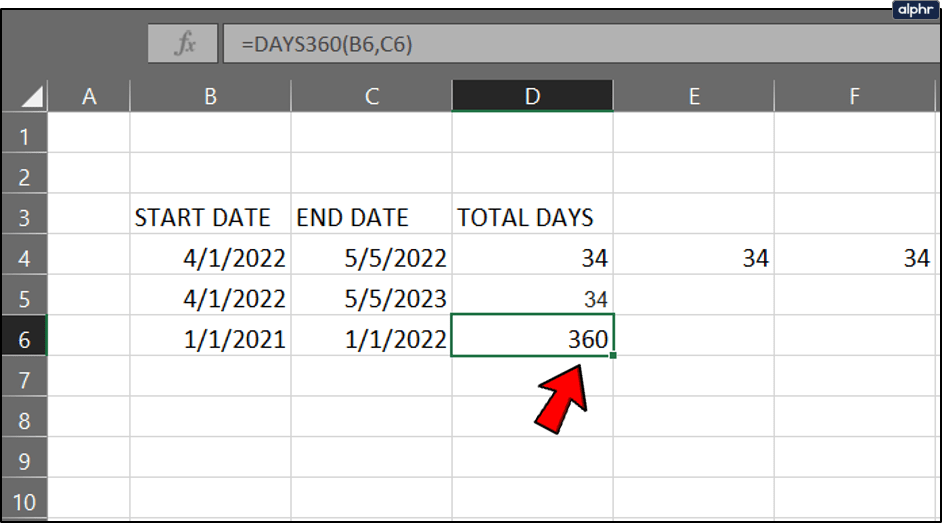## Finding the Difference Between Two Dates using the NETWORKDAYS Function

What if you need to find the difference between any two dates but exclude weekends from the equation? DATEDIF, DATE, and DAYS360 won’t suit such a scenario. NETWORKDAYS is a function that finds the number of days between dates without including any weekends, and it can also factor in extra holidays, such as bank holidays.

So it’s supposed to be a function for project planning. The function’s basic syntax is: =NETWORKDAYS(start_date, end_date, [holidays]).

1. Click on a cell where you want to add the function and Select “Formulas > Date & Time > NETWORKDAYS.”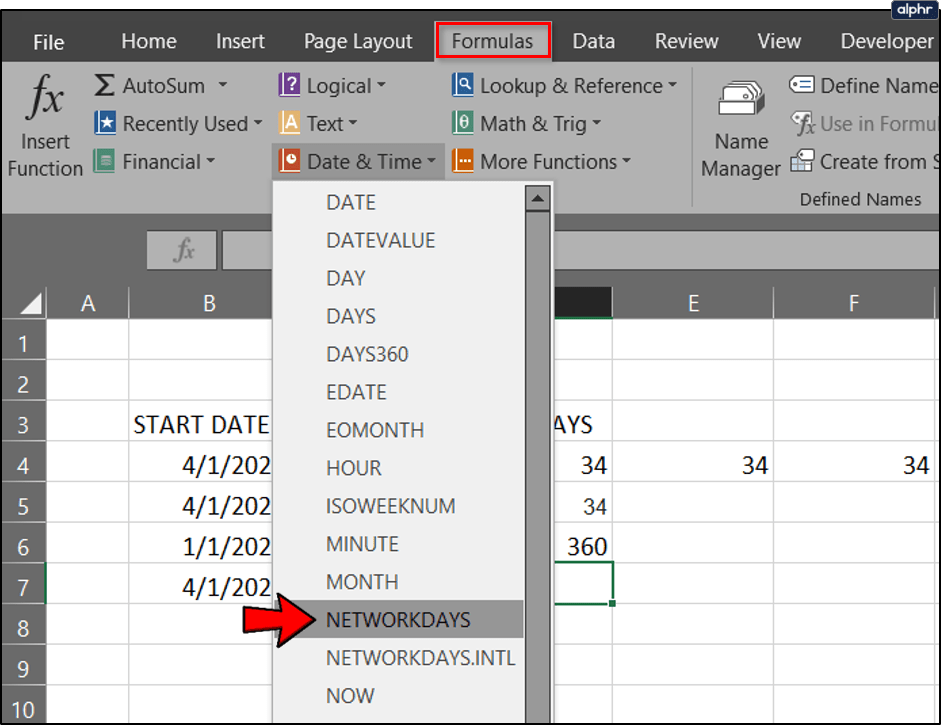2. Type “B7” for the “Start_date” and “C7” for the “End_date” and click “OK.”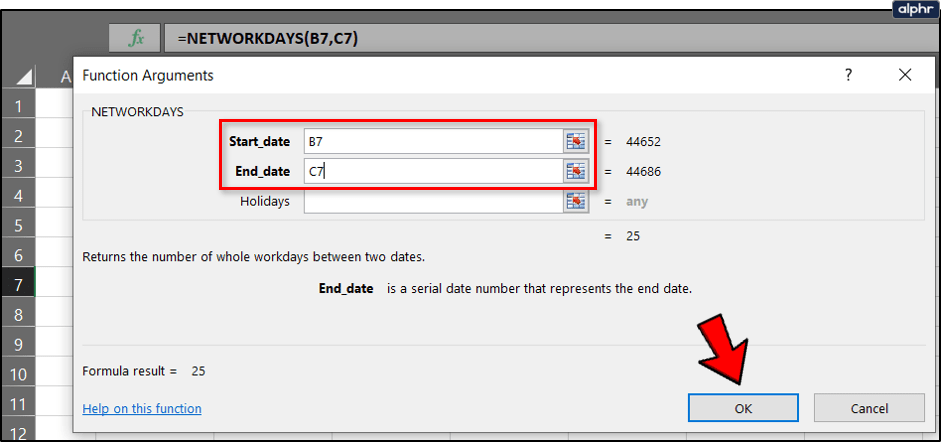3. Using the start and end dates of 4/1/2022 and 5/5/2022, the NETWORKDAYS function returns a value of 25 days between the dates, not counting the weekends. With the weekends included, the total days are 34, as with earlier examples.4. Enter the dates in other spreadsheet cells to include extra holiday days in the function. Press the “Holidays” cell reference button on the NETWORKDAYS function window and select the cells with holiday dates. That will deduct the holidays from the final figure.As you can see, there are numerous ways you can calculate days between start and end dates in Excel spreadsheets. More recent Excel versions also include a DAYS function that you use to find the difference between a couple of dates. Those functions will undoubtedly be helpful for spreadsheets that have lots of dates.

### What does #NUM mean?

When you perform the formulas above and receive #NUM rather than a number, the start date exceeds the end date. Try flipping the dates around and performing the steps again.

## One thought on “How to Calculate Days Between Two Dates in Excel”Ab says:
How many days are there 1/16/2019 through 2/26/2019
The “Days” function returns 41 but if you count I think it’s 42Steve Larner says:
It’s only 42 if you count the initial day, 1/16/2019, but normally, the next day counts as the first day.

Disclaimer: Some pages on this site may include an affiliate link. This does not effect our editorial in any way.

Todays Highlights